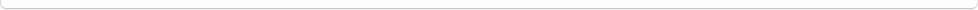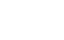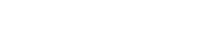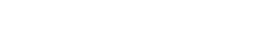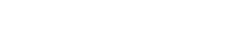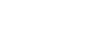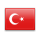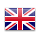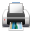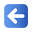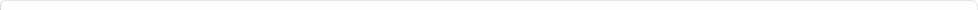Description of Individual Course Units
 Course Unit Code Course Unit Title Type of Course Unit Year of Study Semester Number of ECTS Credits 9900000219 MATHEMATICS - II Compulsory 1 2 2
Level of Course Unit
Short Cycle
Objectives of the Course
This derste; It is aimed to gain knowledge and skills about mathematics in profession.
Name of Lecturer(s)
Dr. Öğr. Üyesi Emin Taner ELMAS
Learning Outcomes
 1 To make applications related to VECTORS, COMPLEX NUMBERS, EQUATIONS AND EQUALITIES 2 To make applications about Matrix, Linear Equation Systems and STATISTICS
Mode of Delivery
Evening Education
Prerequisites and co-requisities
None
Recommended Optional Programme Components
None
Course Contents
1 VECTORS 2 VECTORS 3 COMPLEX NUMBERS 4 COMPLEX NUMBERS 5 COMPLEX NUMBERS 6 COMPLEX NUMBERS 7 EQUATIONS AND EQUALITIES 8 EQUATIONS AND EQUALITIES 9 Matrices 10 Matrices 11 GEOMETRIES 12 Linear Equation Systems 13 Linear Equation Systems 14 STATISTICS
Weekly Detailed Course Contents
 Week Theoretical Practice Laboratory 1 VECTORS X 2 VECTORS X 3 COMPLEX NUMBERS X 4 COMPLEX NUMBERS X 5 COMPLEX NUMBERS X 6 COMPLEX NUMBERS X 7 EQUATIONS AND EQUALITIES X 8 EQUATIONS AND EQUALITIES X 9 MATRIX X 10 MATRIX X 11 GEOMETRY X 12 LINEAR EQUATION SYSTEMS X 13 LINEAR EQUATION SYSTEMS X 14 STATISTICS X
Planned Learning Activities and Teaching Methods
Assessment Methods and Criteria
 Term (or Year) Learning Activities Quantity Weight Midterm Examination 1 100 SUM 100 End Of Term (or Year) Learning Activities Quantity Weight Final Examination 1 100 SUM 100 Term (or Year) Learning Activities 40 End Of Term (or Year) Learning Activities 60 SUM 100
Language of Instruction
Turkish
Work Placement(s)
None